#### A dynamo-like mechanism

Though we favour the previous model based on the action of turbulent magnetic diffusion, let us show that there is also a special kind of dynamo or amplification mechanism that, in contrast with the standard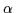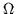, quickly reaches steady state conditions, and that also produces the critical profile of the magnetic field strength.

Let us continue considering azimuthal symmetry. In addition toterms, let us considerterms, i.e. the effect based on a mean value of the quantity <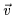.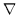×>, non vanishing in turbulence velocity fields, because the Coriolis force introduces order into the chaotic turbulence. With this symmetry the induction equation becomes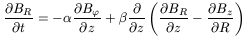(138)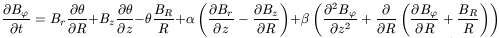(139)(140)

If, as in the previous Section, we set BR = 0, Bz constant, and assume steady state conditions, this equation system reduces to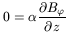(141)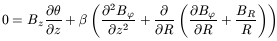(142)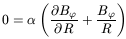(143)

The first equation confirms our previous assumption. The third equation is very illustrative, as, even if= 0, in the absence of significant turbulent magnetic diffusion, we again obtain the critical profile B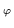= B*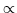. As the first one informs us that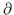B/z, then the second just tells us that this solution is compatible with either Bz = 0, or no vertical differential rotation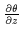= 0. It is important to note that we have obtained the critical profile both withoutand including. In contrast with other dynamos, this mechanism can reach a steady state.